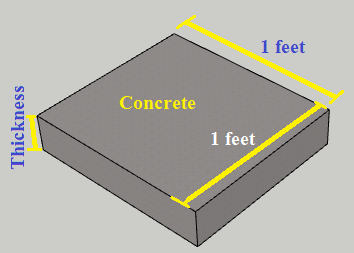# Weight of concrete per square foot Calculation

Concrete is a dense mass which is used for construction purposes. Concrete is a mixture of different ingredients like cement, sand, admixture and aggregate. So, the mass or weight of concrete depends upon the quantity of ingredient mixed to prepare the concrete.

Different grade of concrete has different ratio of ingredient. Like the ratio of Cement : Sand : Aggregate = 1:2:3. It means that if you add one part of cement by volume then you have to add two parts of sand and three parts of aggregate.

In the simillar way, if we take the ratio of ingredients as Cement : Sand : Aggregates = 1:3:6, then we have to add one part of cement with three parts of sand and six parts of aggregate. The ratio of ingredient decide, the strength of concrete.

By the way, according the various test of concrete, the density of PCC ie. plain cement concrete can be taken as 2400 kg/m3 and the density of RCC i.e Reinforced cement concrete can be taken as 2500 kg/m3.

PCC is the concrete which does not contain steel rebar in it, and RCC means the concrete which contains steel bars in it. Now let us see the factors affecting the weight of concrete per square foot.

## Factor affecting weight of concrete per square foot

#### a) Quantity of ingredient

Quantity of ingredients like cement, sand, and aggregate play very important role in the weight of concrete. The density of different ingredients has a different value. So, if we add the higher density ingredient like aggregate, gravel etc then the weight of concrete will obviously increase.

In the same way, if we add lower density ingredients like sand, then the weight of concrete per square foot will decrease.

#### b) Thickness of concrete

We know the weight of concrete is the product of volume and density. So, if we increase the thickness of concrete keeping the area one square foot (i.e Length = 1 foot, Width = 1 foot) then the volume of concrete will increase which results in the increase of weight in concrete and vice versa.

#### c) Insertion of steel rebar in it

Steel has high density than other materials in concrete. So, when we insert steel bars in concrete then the weight of the concrete will increase. The relative density of concrete with steel becomes higher as compared to steel-free concrete.

#### d) Presence of moisture in concrete

Moisture in concrete makes the concrete heavy as compared to the dry concrete. So, we should leave the concrete for up to three months to make it significantly dry.

## How to calculate the weight of concrete per square foot?

Numerical,

Let, us we have a concrete with thickness 6″ (inches). Then what will be the weight of concrete per square foot without steel rebar?

Solution:-

We have, Length of Concrete = 1 foot

Width of concrete = 1 foot

Thickness of concrete = 6 inch (6″) = 0.5 feet

(Since, 1 feet = 0.5 feet)

Now, Volume of concrete = Length x Width x Thickness

Volume = 1 x 1 x 0.5 feet

Volume = 0.5 feet square = 0.0141584 meter cube

[Since, 1 feet cube = 0.0283168 cubic meter]

[We know density of concrete without rebar = 2400 kg/m3]

i.e Density = 2400 kg/m3

Hence, Weight of Concrete = Volume x Density

Weight = 0.0141584 x 2400

= 33.98 Kg = 74.913 Pound = 1198.609 Ounce

#### Convertion Unit

1 Kg = 1000 Gram

1 Kg = 0.00110231 US Ton

1 Kg = 2.20461 Pound

1 Kg = 35.2739 Ounce

If we change any of the factors given above, then the weight of the concrete will be changed.

## Weight of concrete per square foot Calculator